Part 1：Training the Network（训练算法篇），介绍CTC理论原理，包括问题定义、公式推导、算法过程等。Part1 链接
Part 2：Decoding the Network（解码算法篇），介绍CTC Decoding的几种常用算法。Part2 链接
Part 3：CTC Demo by Speech Recognition（CTC语音识别实战篇），基于TensorFlow实现的语音识别代码，包含详细的代码实战讲解。
Part 4：CTC Demo by Handwriting Recognition（CTC手写字识别实战篇），基于TensorFlow实现的手写字识别代码，包含详细的代码实战讲解。
Part 5：Conclusion（总结展望篇），总结CTC算法的理论局限性和适用场景，以及近年来相关的最新研究动态。

# 1 为什么需要CTC解码算法？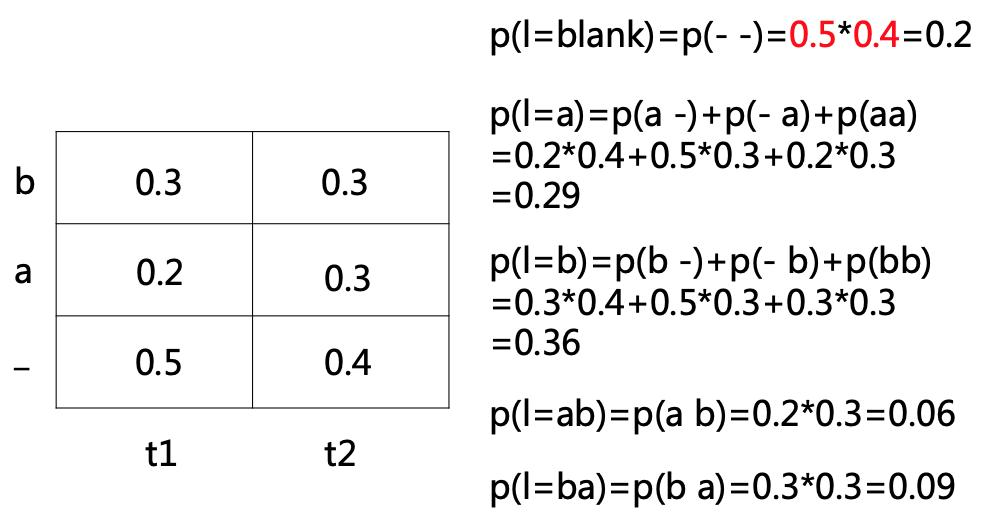# 2 CTC解码算法

## 2.1 CTC Prefix Search Decoding

CTC Prefix Search Decoding本质是贪心算法，每一次搜索都会选取“前缀概率”最大的节点扩展，直到找到最大概率的目标label，它的核心是利用动态规划算法计算“前缀概率”。下面先通过一个简单的例子来介绍CTC Prefix Search Decoding的大致过程，如下图。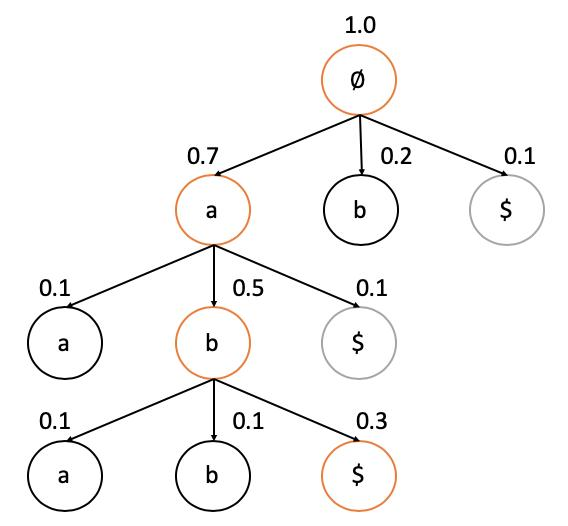（1）初始化最佳序列l^{\ast }为空集，最佳序列概率为 P(l^{\ast } )。把根节点放入到扩展集合中，初始化它的前缀概率为1.0，初始化P(l^{\ast } = 0)
（2）从扩展集合中选取前缀概率最大的节点扩展，扩展子节点a和b，计算a和b的前缀概率（上图中第一层节点a和b的前缀概率分别为0.7和0.2），如果前缀概率大于P(l^{\ast } )则将其加入到扩展集合。同时，计算结束节点的概率（上图中第一层节点\circledS 的概率为0.1），如果结束节点的概率大于P(l^{\ast } )，则将其对应的label设置为最佳序列l^{\ast }，同时更新P(l^{\ast } )
（3）继续搜索，重复步骤2，直到扩展集合为空，即搜索结束，输出最终解码的l^{\ast }和概率P(l^{\ast } )，上图中，最终l^{\ast }=abP(l^{\ast } )=0.3

（a）\gamma-(\rho ,t)定义为t时刻网络输出blank空字符的概率；
（b）\gamma+(\rho ,t)定义为t时刻网络输出非空字符的概率，则\gamma(\rho ,t) = \gamma-(\rho ,t) + \gamma+(\rho ,t)

Y= \left \{ \pi \in L^{n} :\beta (\pi )=\rho \right \}

t时刻的前缀\prime的概率为：

\begin{array}{c}
\gamma (\rho ,t)=\sum P(\pi|x) (\pi \in Y) \\
\gamma+(\rho ,t)=\sum P(\pi|x) (\pi \in Y,\pi_{t}=\rho_{ \left | \rho \right | } ) \\
\gamma-(\rho ,t) = \sum P(\pi|x) (\pi \in Y,\pi_{t} = blank ) \\
\end{array}

\begin{array}{c}
p(\rho |x) = \gamma (\rho ,t) = \gamma- (\rho ,t)+\gamma+ (\rho ,t),p(\rho... |x)= {\textstyle \sum_{s\ne \phi }^{p}}(\rho + s|x)
\end{array}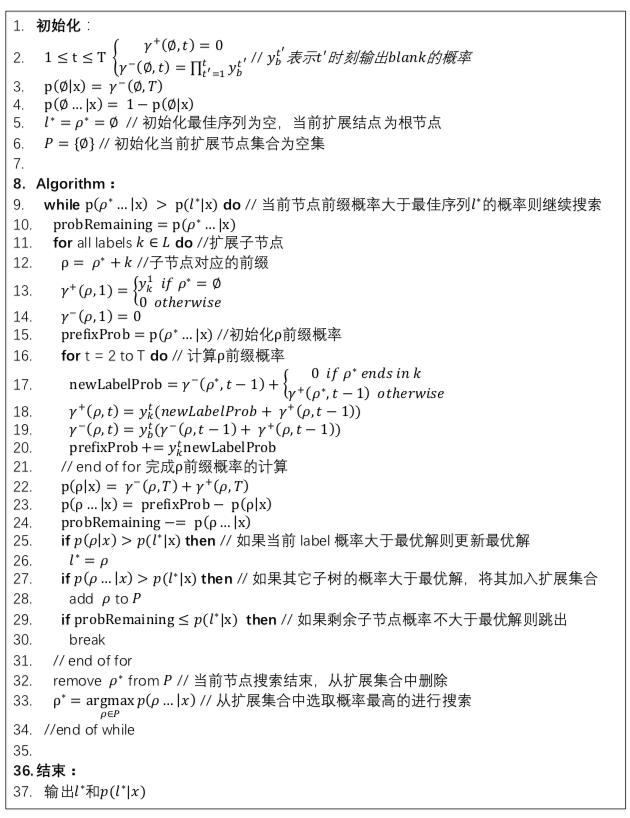\begin{array}{c}
argmax_{l} p(l|x,G)
\end{array}

\begin{array}{c}
p(l|x,G) = \frac{p(l|x)p(l|G)p(x)}{p(x|G)p(l)}
\end{array}

\begin{array}{c}
argmax_{y} p(l|x)p(l|G)
\end{array}

## 2.2 CTC Beam Search Decoding

CTC Beam Search Decoding算法虽然简单，但在实际中应用广泛，我们有必要深入了解它的具体实现细节。Beam Search的过程非常简单，每一步搜索选取概率最大的W个节点进行扩展，W也称为Beam Width，其核心还是计算每一步扩展节点的概率。我们先从一个简单的例子来看下搜索的穷举过程，T=3，字符集为{a, b}，其时间栅格表如下图：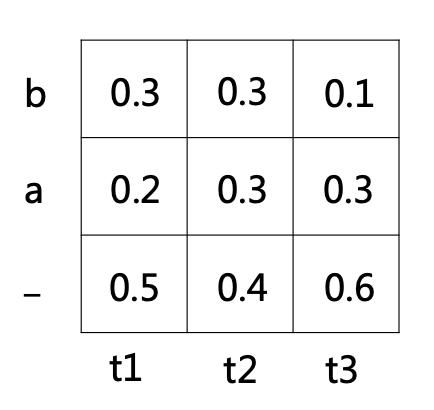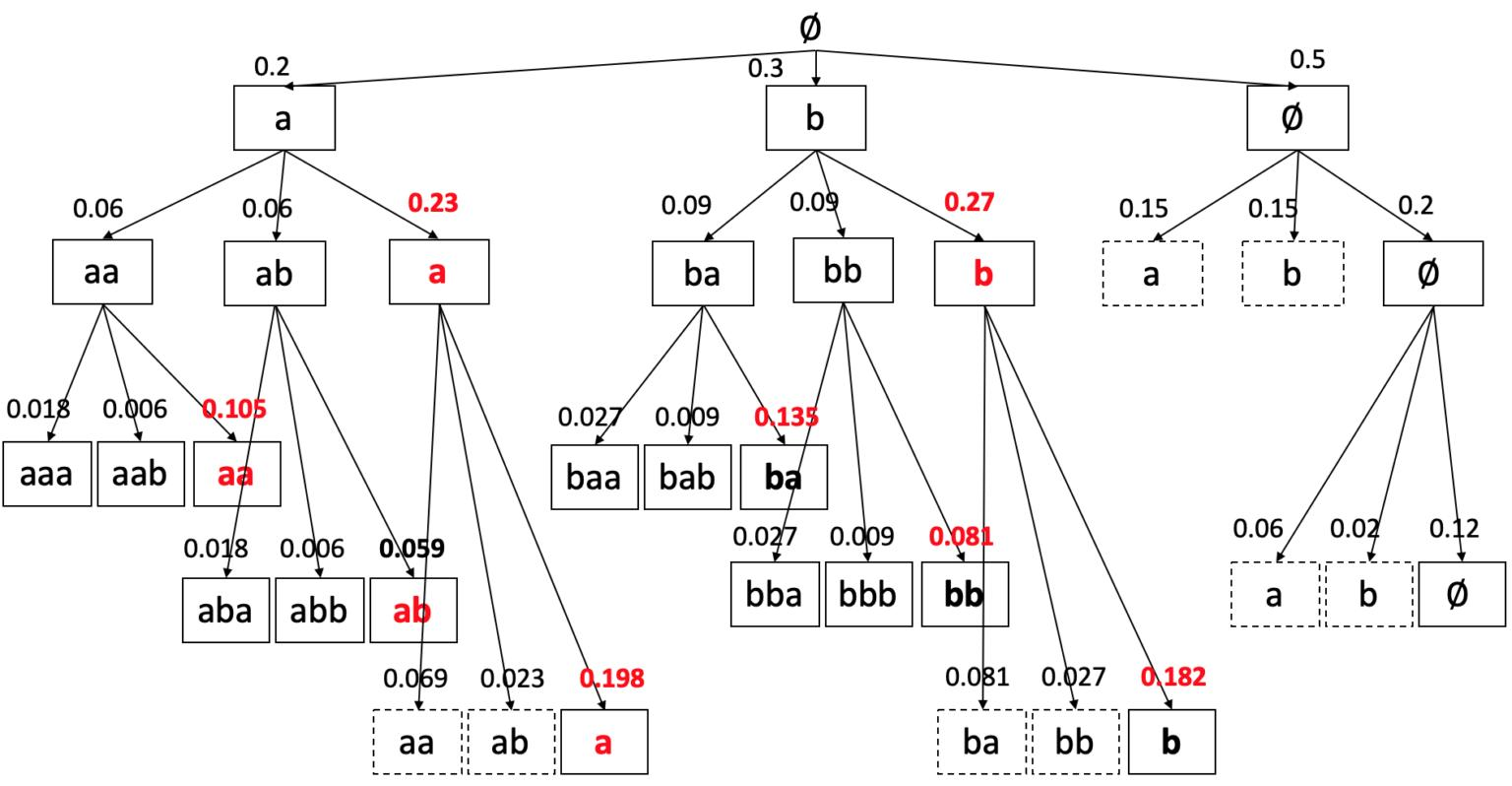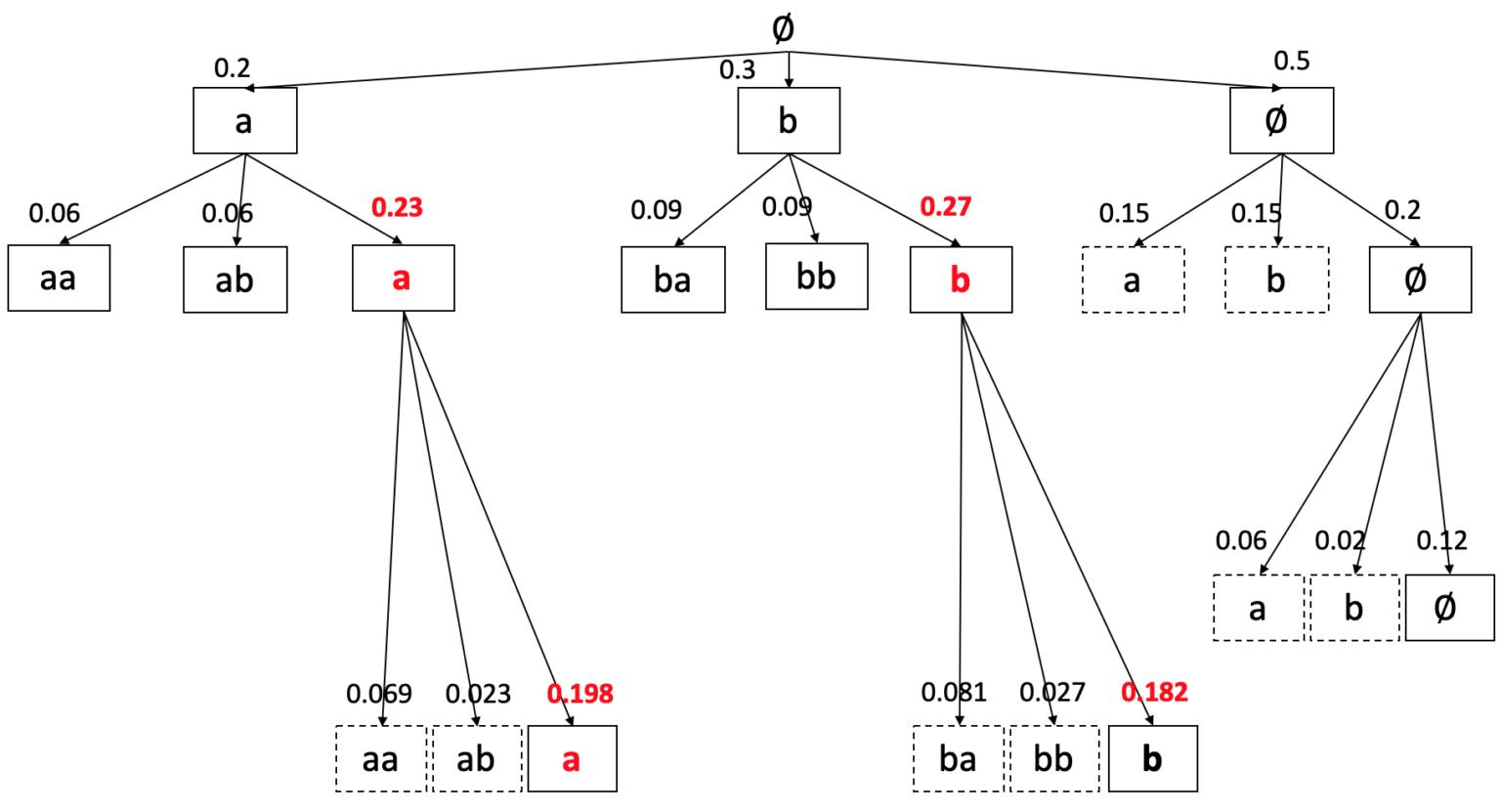（1）定义\hat{\rho}\rho的前继前缀，比如\rho=ab，则\hat{\rho}=a
（2）定义\rho ^{e}为字符串\rho的结尾字符，比如\rho=abc，则\rho ^{e}=c
（3）定义\hat{\rho } ^{e}为字符串\hat{\rho}的结尾字符，比如\hat{\rho}=ab，则\hat{\rho } ^{e} = b

\gamma (\rho ,t)划分为两种情况，
（a）\gamma-(\rho ,t)定义为t时刻网络输出blank空字符的概率；
（b）\gamma+(\rho ,t)定义为t时刻网络输出非空字符的概率，则\gamma(\rho ,t) = \gamma-(\rho ,t) + \gamma+(\rho ,t)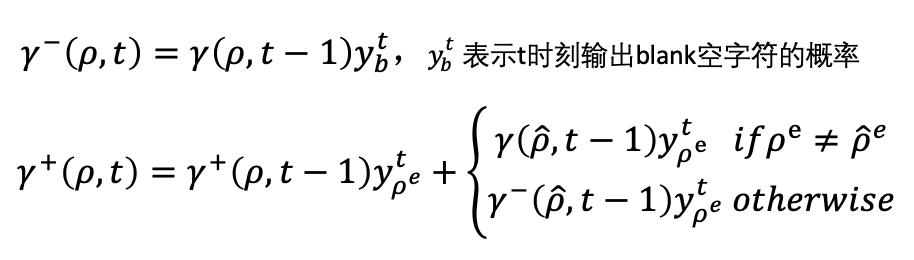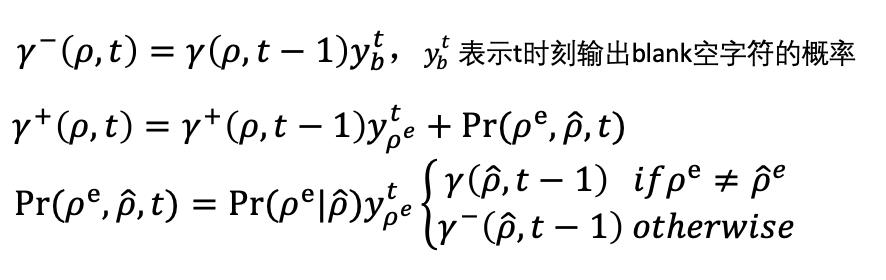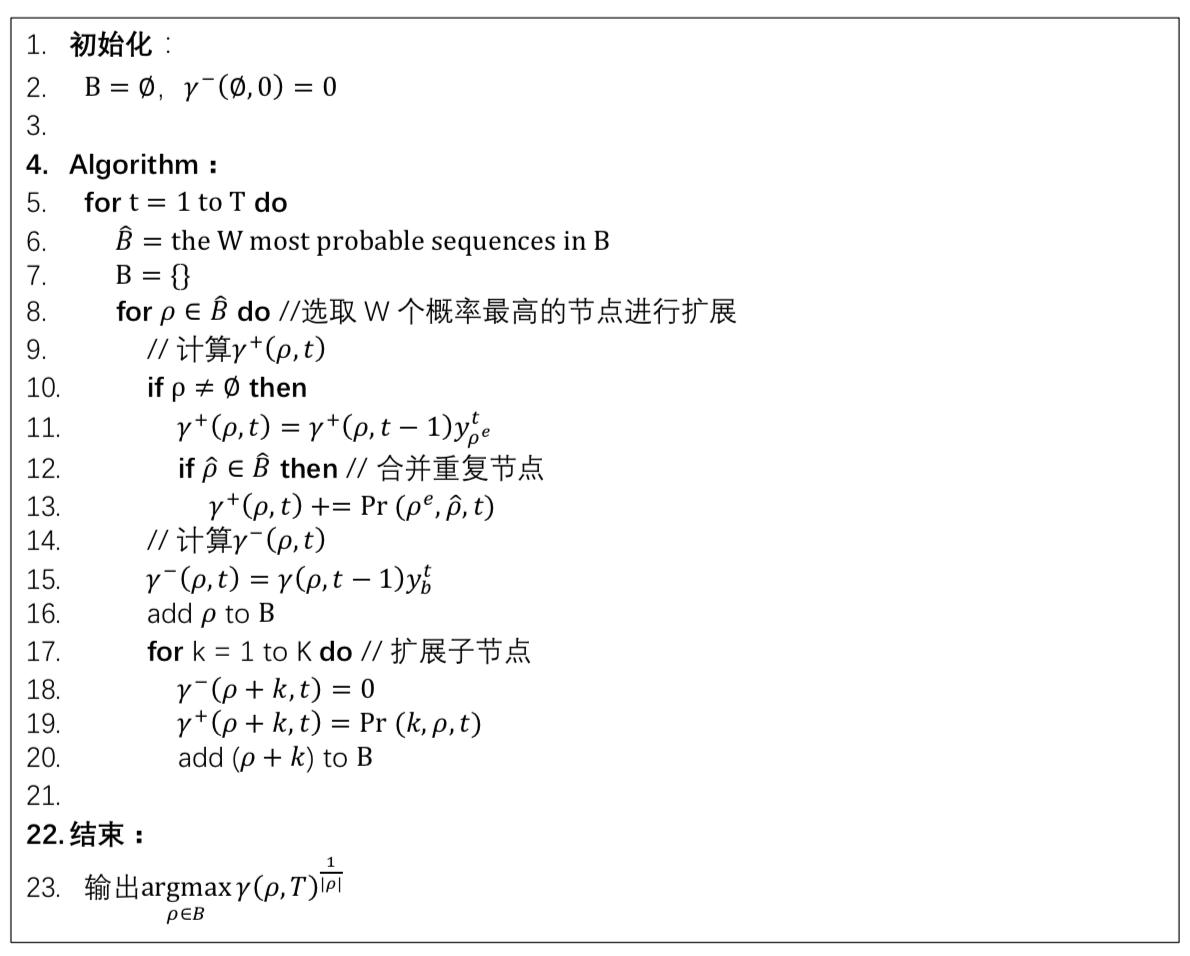1. Graves et al., Connectionist Temporal Classification: Labelling Unsegmented Sequence Data with RNNs. In ICML, 2006. （Graves提出CTC算法的原始论文）
2. Graves et al., A Novel Connectionist System for Unconstrained Handwriting Recognition. In IEEE Transactions on PAML, 2009.（CTC算法在手写字识别中的应用）
3. Graves et al., Towards End-to-End Recognition with RNNs. In JMLR, 2014.（CTC算法在端到端声学模型中的应用）
4. Alex Graves, Supervised Sequence Labelling with Recurrent Neural Networks. In Studies in Computational Intelligence, Springer, 2012.（ Graves 的博士论文，关于sequence learning的研究，主要是CTC）